International
Tables for
Crystallography
Volume D
Physical properties of crystals
Edited by A. Authier

International Tables for Crystallography (2006). Vol. D, ch. 1.1, p. 15

## Section 1.1.4.6.2.1. Introduction

A. Authiera*

aInstitut de Minéralogie et de la Physique des Milieux Condensés, Bâtiment 7, 140 rue de Lourmel, 75015 Paris, France
Correspondence e-mail: aauthier@wanadoo.fr

#### 1.1.4.6.2.1. Introduction

| top | pdf |

If one takes as the system of axes the eigenvectors of the operator A, the matrix is written in the form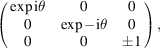where θ is the rotation angle,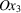is taken parallel to the rotation axis and coefficientis equal to +1 or −1 depending on whether the rotation axis is direct or inverse (proper or improper operator).

The equations (1.1.4.1)can then be simplified and reduce to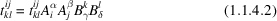(without any summation).

If the product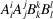(without summation) is equal to unity, equation (1.1.4.2)is trivial and there is significance in the component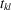. On the contrary, if it is different from 1, the only solution for (1.1.4.2)is that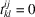. One then finds immediately that certain components of the tensor are zero and that others are unchanged.